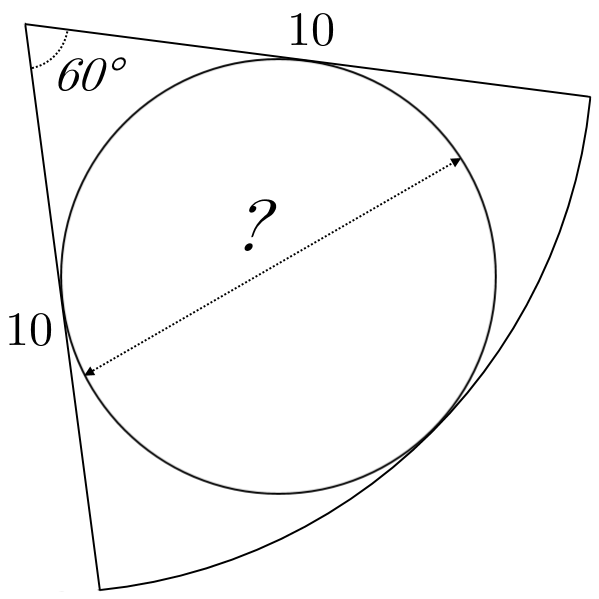# Circle-ception: The next level

Geometry Level 4A sector of a circle of radius $10$ and subtended angle $60°$ has a circle inscribed in it - its diameter can be written as $\frac { a }{ b }$, where $a$ and $b$ are co-prime integers.

Find the value of$\left\lceil \frac { { a }^{ 2 }b^{ 2 } }{ a+b } \right\rceil$

×